Reach Us+44-1522-440391
Surface Thermodynamics Approach to <em>Mycobacterium tuberculosis</em> (MTB) Human Sputum Interactions | OMICS International
Journal of Bioengineering & Biomedical Science

Like us on:

All submissions of the EM system will be redirected to Online Manuscript Submission System. Authors are requested to submit articles directly to Online Manuscript Submission System of respective journal.

Surface Thermodynamics Approach to Mycobacterium tuberculosis (MTB) Human Sputum Interactions

Chukwuneke JL*, Achebe CH and Omenyi SN

Mechanical Engineering Department, nnamdiazikiwe University, Awka, Nigeria

*Corresponding Author:
Chukwuneke JL
Mechanical Engineering Department
nnamdi Azikiwe University, Awka, Nigeria
Tel: +234 08033574353
E-mail: [email protected]

Received Date: August 10, 2015; Accepted Date: September 30, 2015; Published Date: October 10, 2015

Citation: Chukwuneke JL, Achebe CH, Omenyi SN (2015) Surface Thermodynamics Approach to Mycobacterium tuberculosis (M-TB) – Human Sputum Interactions. J Bioengineer & Biomedical Sci 5:167.doi:10.4172/2155-9538.1000167

Copyright: © 2015 Chukwuneke JL, et al. This is an open-access article distributed under the terms of the Creative Commons Attribution License, which permits unrestricted use, distribution, and reproduction in any medium, provided the original author and source are credited.

Visit for more related articles at Journal of Bioengineering & Biomedical Science

Abstract

This research work presents the surface thermodynamics approach to M-TB/HIV-Human sputum interactions. This involved the use of the Hamaker coefficient concept as a surface energetics tool in determining the interaction processes, with the surface interfacial energies explained using van der Waals concept of particle interactions. The Lifshitz derivation for van der Waals forces was applied as an alternative to the contact angle approach which has been widely used in other biological systems. The methodology involved taking sputum samples from twenty infected persons and from twenty uninfected persons for absorbance measurement using a digital Ultraviolet visible Spectrophotometer. The variables required for the computations with the Lifshitz formula were derived from the absorbance data. The Matlab software tools were used in the mathematical analysis of the data produced from the experiments (absorbance values). The Hamaker constants and the combined Hamaker coefficients were obtained using the values of the dielectric constant together with the Lifshitz equation. The absolute combined Hamaker coefficients A132abs and A131abs on both infected and uninfected sputum samples gave the values of A132abs = 0.21631×10-21 Joule for M-TB infected sputum and Ã132abs = 0.18825×10-21 Joule for M-TB/HIV infected sputum. The significance of this result is the positive value of the absolute combined Hamaker coefficient which suggests the existence of net positive vanderwaals forces demonstrating an attraction between the bacteria and the macrophage. This however, implies that infection can occur. It was also shown that in the presence of HIV, the interaction energy is reduced by 13% conforming adverse effects observed in HIV patients suffering from tuberculosis.

Keywords

Absorbance; Dielectric constant; Hamaker coefficient; Lifshitz formula; Macrophage; Mycobacterium tuberculosis; van der Waals Forces

Introduction

The World Health Organization (WHO) declared tuberculosis (TB) as a global emergency in 1993. Unfortunately, the efforts made by the Stop TB Strategy were not enough to impede the occurrence of 1.3 million deaths in 2009 . However, WHO estimates that the number of cases per capita peaked at 2004 and is slowly falling . Nonetheless, the battle against TB is far from being over, since Mycobacterium tuberculosis (the main causative agent of TB) proved to be highly adaptive  and capable of evading the current strategies for treatment of about half million cases of multi-drug-resistant TB (MDR-TB) that were reported in 2007, including cases of extensively drug-resistant TB (XDR-TB) , and the more recently reported totally drug-resistant strains (TDR-TB) [4,5].

Several reviews so far have been reported the incidences of TB cases, a particular report surveyed that out of 134 countries only 35 showed declination of cases of around 5% per year based on per capita rate . This survey considered the data from 1998 to 2007. Different surveillance analysis and mathematical modeling studies recommended reduction of TB incidences per capita is around 1% per year, further suggesting diminution of cases by 2015. It is being predicted that growth of the world population of approximately 2% per year may be an important reason for increment of TB cases . All these previous reports showing the presence of lacunae in the existing management approaches for TB and the inadequate effectiveness of public health systems, with special reference to underdeveloped countries. In spite of the availability of anti-TB drugs developed over the last five decades, one-third of the world’s population retains a dormant or latent form of Mycobacterium tuberculosis (M-TB).

In addition, drugs such as Rifampicin have high levels of adverse effects making them prone to patient incompliance. Another important problem with most of the anti-mycobacterials is their inability to act upon latent forms of the bacillus. To compound the problem further, the complex (vicious) interactions between the HIV and TB makes the treatment of co-infected patients even more challenging [5,7].

In this study, M-TB is conceptualized as particle dispersed in a liquid (the sputum) and interacting with another particle (macrophage). The bacterium attaches itself on the surface of the macrophage cell before penetrating and attacking it. If the surface of the macrophage cell is such that it will repel the bacteria, access of the bacteria into the alveoli of the cell would have been denied. Thus the initial actions actually take place on the surface of the cell and of the bacteria.

It is a well-known fact that surface property determination of interacting particles lead to the further understanding of the mechanism of interactions. A common area of contact is established once two particles meet each other. In such process, a certain portion of each particle gets displaced through work. Work responsible for the displacement of a unit area is known as surface free energy. The consecutive impact on the surface is known as surface thermodynamic effects. To attain the equilibrium such impacts are changed in a slow pace. In this particular study similar concepts have been implemented to characterize the M-TB – macrophage interactions with the sputum as the intervening medium.

Related works are in the phagocytosis of bacteria platelets advanced by Neumann et al. , van Oss et al.  and Absolom et al. , from surface thermodynamics point of view. Their works considered the relationship between free energy of engulfment and number of bacteria ingested. It is against this backdrop that this study explores a novel and rare approach using surface thermodynamics to seek a way forward in the research on the topic of Mycobacterium tuberculosis human system interaction.

Theoretical Considerations

Thermodynamic approach to particle-particle interaction

Suppose in a certain case, the bacterium, M-TB has been considered as a particle coming in vicinity to the macrophage cell which is further considered as another particle. In the consecutive step one particle (the bacterium) attaches itself on the surface of the other (the macrophage) under a specific condition where the macrophage is dispersed in sputum (Figure 1).

Expression of the thermodynamic free energy associated with adhesion process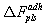provided in Figure 1 is represented as follows :

ΔFplsadh (do) = γps – γpl - γsl (1)

In this particular expression, ΔFadh represents the integrated free energy of adhesion considered from infinity to the equilibrium where separation distance is do. In this equation, P, S represents bacteria and macrophage cell respectively and L signifies the sputum. In the right hand side of the equation, γps expresses the interfacial free energy between P and S. γpl represents the same for P and L, whereas, γsl provide the same information between S and L.

Successful bacterial infiltration in the macrophage cells will occur through the engulfment of the bacteria requiring net free energy, which is represented as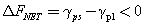In this equation, rejection of the bacteria by the macrophage will be represented, if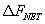becomes greater than zero. It has been reported that solid-liquid interfacial tension (γps) plays a crucial role in several scientific problems. In certain situations, explanation of several scientific phenomena, such as, cell adhesion, protein adsorption, phagocytosis, etc. were extensively understood through thermodynamic models developed on the basis of interfacial tensions . Except fluid-fluid interfacial tensions, direct measurement of interfacial tensions containing a solid phase are cumbersome .

The most routine strategy for these interfacial tensions calculation is through interpretation of contact angle data.

Properly prepared solid surfaces helps in measuring the contact angles, hence, aiding in estimating solid-liquid (γsl), and solid-vapour (γsv) interfacial tensions [12,14].

The solid-liquid interfacial tensions could be expressed as solid and liquid vapour to estimate γsl and γsv from contact angle source following the provided equations :

γsl = f (γsvlv ) (2)

Introducing the contact angle to this equation results in Young’s equation;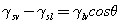(3)

An explicit expression for this relation is given by Neumann ;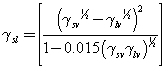(4)

Where θ is the Young contact angle and defined as follows;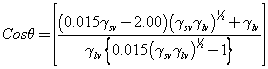(5)

The equation of state, eqn. (4), in conjunction with eqn. (3) can be used to determine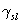and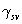from contact angle data θ.

There are available several other interfacial tension equations based on surface tension components approach , in terms of the dispersion and polar interactions.

If in this study, it was assumed that the interfacial free energies are dispersive, then the polar contributions to the particle interfacial tension play no role in determining the interfacial tensions. The total free energy of adhesion will be given solely by the dispersion interactions, and can be obtained from Lifshitz theory considerations. The advantage of using Lifshitz-van der Waals interactions instead of the interfacial tension components equation was stated earlier. The calculation of dispersion interactions from Lifshitz theory is independent of contact angle data and these interactions are not approximated by geometric means as is the case when surface tension component equations are used .

Lifshitz theory

A system containing two planes could be considered for computing the free energy of interaction. This can be done for semi-infinite, parallel bodies belonging to material 1 and 2 isolated by material 3, bearing thickness L (Figure 2) provided in the following section [18,19]. This is calculated by the following equation: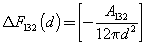(6)

Figure 2: Schematic representation of interaction of two solid bodies, depicted by 1 and 2 which are eventually isolated by d, liquid 3.

In this, A132refers to the Hamaker coefficient for a respective system (Figure 2).

Considering nominal isolation distance d0, and Eqn. (6) as valid for such a small distance, the Hamaker coefficient should be expressed as [18,19]: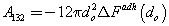(7)

The Hamaker coefficient A132 for the interactions between two different bodies in a liquid can be calculated from Eqn.(7) once the free energy of adhesion between the two bodies is known or through the pair-wise additivity approach as originally proposed by Hamaker  or by the macroscopic approach of Lifshitz .

Influence of neighbouring atoms remains major hurdle during the pair-wise summation computing between various molecular interactions. In case of highly disperse media such influence is insignificant, for instance, gases whereas for condensed media it is important .

As the actual material atomic structures are overlooked, the Lifshitz method is suitable in certain cases. In this method, bulk material properties are considered for calculation of interactions between the macroscopic bodies. Properties like refractive indices and dielectric permittivity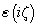are considered for such calculations. Dielectric permittivity represents the microscopic polarizability as a manifested macroscopic property for the constant atoms belonging to certain materials. The Hamaker coefficient represents the macroscopic resultant for the interactions happened due to the atom polarizations in a material .

Following Lifshitz theory, the Hamaker coefficient is represented as follows: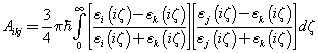(8)

Where, εj(iζ) refers to the dielectric constant of a specific material j, this is considered through the imaginary i, frequency axis (iζ), ? is planck’s constant. In this context, the evaluation of Eqn. (6) should result in equivalent value with the thermodynamic free energy of adhesion, provided in Eqn. (1). The molecular contact was maintained at (d=0). Interestingly, constituent molecule numbers are of finite size and for that it is not possible to attain d=0 for two macroscopic surface. Therefore, whenever the surfaces attain a distance do, molecular contacts are considered. The divergences according to Lifshitz theory are eliminated by the parameter do.

Thus, the Hamaker coefficient or the Lifshitz-van der Waals constant A132 may result in negative. In certain condition, the contact between the interacting particles will be hampered due to the repulsive (electrostatic) force originated. The resultant effect in certain cases remains as repulsion instead of attraction for the considered particles. The Hamaker coefficient and the interfacial free energies are connected through the following equation: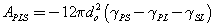(9)

This equation has been derived through combining Eqn. (7) with Eqn. (1).

For the issue of self-interaction of a particle Eqn. (8) should be considered;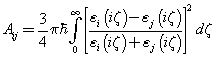(10)

Therefore, the systems under consideration follows the below equations: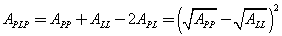(11)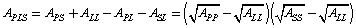(12)

Materials and Methods

To determine the combined Hamaker coefficient using the Lifshitz theorem of eqn. (8), there is a need to evaluate the dielectric constant ε of that equation. This can only be done through the measurement of the absorbance for each sample of infected M-TB sputum, uninfected M-TB sputum and TB/HIV co-infected sputum.

Sample collection

This research work involved collection of Sputum samples from twenty TB infected, twenty uninfected and twenty HIV/TB co-infected persons. The collected sputum samples were screened to determine the infection status using GeneXpert thus giving a total of sixty sputum samples from different individuals. The sputum samples were collected from Anambra State University Teaching Hospital (ANSUTH) Awka (formerly General Hospital Awka). Spot Specimens were used to ensure the freshness of the collected Sputum samples and to avoid the samples becoming lysed (spoilt). Storage facilities like refrigerators were also used to ensure that the samples were healthy enough so as to obtain good results.

Sample preparation

The collected samples were loaded into GeneXpert Cartridge to determine if the sample is mycobacterium TB positive or negative. This helped to obtain and separate the positive and negative M-TB. The glass slides were prepared and smeared with the samples for absorbance measurements. The slide preparations and sample smearing were done at the same laboratory with proper safety measures to avoid being infected.

The glass slide of 25.4 mm × 76.2 ×1.2 mm was used for the preparation of test surfaces. A dropper was used to draw each of the Sputum samples from the container and smeared carefully on a slide to ensure even distribution of the sputum samples on the slides. Three slides were prepared for each of the twenty sputum samples and smeared with the samples for absorbance measurements. The slide preparations and sample smearing were done at the same laboratory (Chest Clinic/ Laboratory, Anambra State University Teaching Hospital, Awka). The samples were allowed to dry naturally at room temperature because exposing the prepared slides to the sun is likely to cause oxidation and the surface energy might be increased unconditionally. All the well prepared and dried surfaces were covered with microscopic cover slip, ready for the experiment.

Measurements

Absorbance measurements were done on all the positive and negative sputum components of all sixty samples (TB infected, TB uninfected, TB/HIV co-infected and macrophage sputum samples). A digital Ultraviolet Visible Spectrophotometer (UV/ VIS MetaSpecAE1405031Pro) was used in the measurements. The measurements of absorbance and transmittance were done at Bioengineering Labouratory of the department of Mechanical Engineering, Nnamdi Azikiwe University, Awka. The absorbance values of the samples were measured over a range of wavelength spanning between 230 and 950 Å. The data collected were used in calculating the Hamaker coefficients using the Lifshitz formula.

Results and Analysis

Results

Figure 3 shows an interesting pattern for M-TB positive sputum. The absorbance of the individual twenty M-TB infected sputum samples steeply increased as the wavelength increased until a critical wavelength of 320 Å, where the peak value was attained. A further increase in the wavelength saw at first a sudden and latter a gradual decrease in the absorbance values. This peak value falls within the visible range of ultraviolet radiation which is between 300 – 600 Å. The peak values of absorbance range between 0.2918 and 0.7877 (0.2918 ≤ ? ≤ 0.7877). These values are listed for each sample in Table 1. It is interesting though, that at the lower wavelengths of between 230 – 290 Å some negative absorbance values were recorded.

Figure 3: Variation of Absorbance, ? with Wavelength, λ for Twenty Samples of M-TB Infected Sputum.

Sample Type Wavelength, λ(Å) Peak Values Absorbance, ? Peak Values
M-TB Positive M-TB Negative M-TB/HIV Co-infected M-TB/HIV Uninfected Mean Values
Sputum   320 0.2918 – 0.7877 --- --- --- 0.4588 ± 0.1468
--- 0.2657 – 1.2501 --- --- 0.6244 ± 0.3545
290 --- --- 0.0231 – 0.0498 --- 0.0379 ± 0.0108
--- 0.2657 – 1.2501 0.6244 ± 0.3545
Macrophage   320 0.0206 – 0.0736   --- --- 0.0496 ± 0.0116
--- 0.0478 – 0.1148 --- --- 0.0784 ± 0.0206
290 --- --- 0.0152 – 0.0637 --- 0.0456 ± 0.0106
--- --- --- 0.0478 – 0.1148 0.0784 ± 0.0206

Table 1: Comparison between Peak Absorbance values of M-TB Positive, M-TB/HIV Positive and Negative Sputum Components respectively.

Figure 4 shows a similar pattern as that of Figure 3 with the peak value occurring at the wavelength of 320 Å which corresponds exactly with that of the Figure 3. However, the peak absorbance values are of the range 0.0206 and 0.0736 (0.0206 ≤ ? ≤ 0.0736). It is interesting though, that at the lower wavelengths of between 230 – 260 Å some negative absorbance values were recorded (Table 1).

Figure 4: Variation of Absorbance, ? with Wavelength, λ for Twenty Samples of M-TB Infected Macrophage.

Figure 5 reveals an interesting pattern for M-TB/HIV positive Sputum. The absorbance of the respective twenty M-TB/HIV coinfectious sputum samples systematically increased as the wavelength increased until a critical wavelength of 290 Å, where a peak value was accomplished. A further increase in the wavelength saw at first a steady decrease in absorbance until a minimum was attained. As wavelength increases, a progressive increase in absorbance values was recorded and latter a gradual decrease follows. These peak values of absorbance fall within the visible range of the ultraviolet radiation which is between 300 – 600 Å. The peak values of absorbance range between 0.0231 and 0.0498 (0.0231≤ ? ≤ 0.0498), (Table 1) except sample 17 which showed a steady increase as wavelength increased until 410 Å and steeply decreases as wavelength increases; the value of absorbance recorded is obviously a faulty one, this could be explained against the possibility of some experimental error or as a result of some sputum related disease conditions like diabetes, Hepatitis etc. it is interesting though, that at the lower wavelengths of between 230 – 260 Å some negative absorbance values were recorded.

Figure 5: Variation of Absorbance, ? with Wavelength, λ for Twenty Samples of M-TB/HIV Co-infected Sputum.

Figure 6 followed similar pattern as that of Figure 4 with the peak values occurring at the wavelength of 290 Å. However, the peak absorbance values are of the range 0.0152 and 0.0637 (0.0152 ≤ ? ≤ 0.0637) (Table 1). Sample 15 showed a marked departure in its response from the rest until the wavelength of 560 Å and latter decreased systematically as wavelength increased. The continuous increase and latter decrease in the absorbance value recorded is clearly a defective one. This could be explained against the possibility of some experimental or machine error or as a result of some disease related conditions like diabetes, Hepatitis etc.

Figure 6: Variation of Absorbance, ? with Wavelength, λ for Twenty Samples of M-TB/HIV Co-infected Macrophage.

The peak value of the absorbance for M-TB negative sputum of Figure 7 was obtained at the wavelength of 320 Å and ranges as follows 0.2657 ≤ ? ≤ 1.2501 (Table 1). The reaction here also follows the earlier patterns with the various twenty samples showing moderately conformed characteristics. An exception to the rule is found with sample 19 which showed a drop down decrease from the pattern exhibited by the rest of the samples. This again could be explained against machine error.

Figure 7: Variation of Absorbance, ? with Wavelength, λ for Twenty Samples of M-TB Negative Sputum.

Figure 8 for the samples of M-TB Macrophage negative sputum reveals similar characteristics as their counterparts, however with the peak values occurring at 320 Å. The absorbance values at this peak point are of the magnitude between 0.0478 and 0.1148 (0.0478 ≤ ? ≤ 0.1148) (Table 1).

Figure 8: Variation of Absorbance, ? with Wavelength, λ for Twenty Samples of M-TB Macrophage Negative.

Comparison between the peak absorbance values of M-TB positive, M-TB/HIV positive and negative sputum components

From results presented in Figures 3-8 and summarized in Table 1, it could be seen that the peak absorbance values of the various sputum samples and components vary in magnitude revealing the notable effect of the bacteria on them. The comparison between the positive and negative samples of the macrophages is imperative to this research. This is because Mycobacterium tuberculosis actually attacks the macrophages by attaching itself to the macrophage cells. Table 1 reveals also the degree of variation between similar infected and uninfected sputum components at a glance for a clearer understanding (Table 1).

The difference between the peak absorbance values of M-TB positive, M-TB/HIV co-infected and negative sputum components respectively is an indication of how the bacteria affects the properties of the macrophage cells. The trend is such that the mean absorbance peak values of M-TB negative sputum samples are reduced by infection from 0.6244 ± 0.3545 to 0.4588 ± 0.1468 by a factor of about 26.5%. In M-TB macrophage samples, the reduction is from 0.0784 ± 0.0206 to 0.0496 ± 0.0116, a factor of about 36.7%. While in M-TB/HIV coinfected macrophage samples, the reduction is from 0.0784 ± 0.0206 to 0.0456 ± 0.0106 by a factor of about 41.8%. Comparing the mean absorbance peak values of M-TB positive sputum samples and the mean absorbance peak values of M-TB/HIV co-infected sputum samples; the results of the mean absorbance peak values reveal that the mean absorbance peak value of the M-TB/HIV co-infected samples is generally reduced as compared to that of the mean absorbance peak values of the M-TB positive sputum samples (Table 1). The macrophages are of particular interest to this research since the bacteria attacks this T-cells component which serves as receptor cells. Comparing the mean absorbance peak values of M-TB positive macrophage samples and the mean absorbance peak values of M-TB/HIV co-infected macrophage samples; the results of the mean absorbance peak values reveal that the mean absorbance peak values of the M-TB/HIV co-infected samples is generally reduced by infection from 0.0784 ± 0.0206 to 0.0456 ± 0.0106 by a factor of about 41.8% as compared to that of the mean absorbance peak values of the M-TB positive macrophage sample with a factor of about 36.7%. It could be seen that the reduction between the peak values absorbance (mean) of the sputum component is such that the macrophages reduced from M-TB to M-TB/HIV by a factor of about 8.1%. The reduction in the absorbance of the M-TB/HIV infected sputum samples reveals the role of the bacteria in drastically affecting the surface properties of the infected macrophage cells and specimens.

Computation of absolute hamaker coefficients

To be able to use the absorbance data to calculate the Hamaker coefficients using the Lifshitz theorem of eqn. (10), there is a need to evaluate the dielectric constant ε of the equation. Some relevant equations are required as presented below. From the information of light absorbance, reflection and transmittance, it could be seen that;

? + T + R = 1 (13)

Where; ? is absorbance, T is transmittance, and R is reflectance. Also, from the information of light absorbance and transmittance;

T = exp-? (14)

With the values of ? determined from absorbance experimental results, and substituting the values of ? into eqn. (14) to obtain T, R could easily be derived by substituting the values of ? and T into eqn. (13).

To find a value for the refractive index, n employing the mathematical relation ;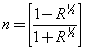(15)

A value for the extinction coefficient, k is obtained from the equation;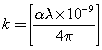(16)

Where; α is the absorption coefficient defined as follows;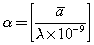(17)

Substituting the value, a of Eq. (17) into Eq. (16);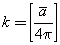(18)

The dielectric constant, ε could thus be given by the formula 

For the real part;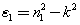(19)

For the imaginary part;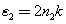(20)

With these values, it is possible to determine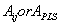using the relevant equations.

Eqn.(10) was used to obtain for each interacting system,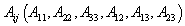by approximate change of variables. MATLAB computation tools were used. This involved the numerical integration of eqn.(10) for each wavelength from 230 to 950 for all the twenty samples in each category. The results are given on Table 2.

M-TB M-TB/HIV
Variable (×10-21Joule) Infected Sputum Uninfected Sputum Variable
(×10-21 Joule)
Infected Sputum Uninfected Sputum
Absolute Value Absolute Value Absolute Value Absolute Value
A11 --- 0.94188 Ã11 --- 0.94188
A22 0.96068 --- Ã22 0.97862 ---
A33 0.23067 0.42470 Ã33 0.28812 0.42470
A132 0.21631 --- Ã132 0.18825 ---
A131         --- 0.10165 Ã131 --- 0.10165
A232 0.24986 --- Ã232 0.20474 ---

Table 2: Values of the Hamaker Constants and Hamaker Coefficients for the Infected and Uninfected Samples.

A33, which serves as the energy of sputum as an intervening medium, is seen in M-TB data to be reduced by infection from 0.4247×10-21 J to 0.23067×10-21 J by a factor of about 45.7%. In M-TB/HIV co-infection, the reduction is from 0.4247×10-21 J to 0.28812×10-21 J, a factor of about 32.2%. The reduction is lower in M-TB/HIV co-infection probably because of the interaction between HIV and TB.

For the combined Hamaker coefficient, the value is 0.21631×10-21 J for M-TB and 0.18825×10-21 J for M-TB/HIV. This result is as expected. HIV has the tendency to reduce the energy on the surface of a given material, in this case by about 13%, conforming adverse effects observed in HIV patients with tuberculosis. Note that the values of A132 are all positive showing that attraction exists between the macrophage and the TB particles. The effect of the infection can only be abated if a drug, in the form of additive is added that can change the value of A132 to negative under that condition, mutual repulsion will occur and it will be expected that, in principle, the TB bacteria will not attack the macrophage.

Conclusion

The positive value of the absolute combined Hamaker coefficient A132=0.21631×10-21 Joule and Ã132 = 0.18825×10-21 Joule obtained for both the M-TB positive and M-TB/HIV positive samples are a confirmation that the sputum samples were actually infected. The absolute Hamaker coefficient A131=0.10165×10-21 Joule gives the interaction energy among the macrophage cells in the sputum while A232 is the interaction energy among the TB particles in the sputum (i.e. A232 is the energy of interaction among the TB particles or among the TB/HIV particles in sputum). A232 for TB/HIV co-infection is less than that for TB alone. Reduction in energy in the presence of HIV confirms the adverse effect when TB and HIV occur simultaneously in a patient. Reduction in energy leads to reduction in CD4 in HIV patient and hence greater prospect for death. This is so since a positive Hamaker value for any interacting system implies an attraction between the interacting bodies or particles while a negative Hamaker coefficient means repulsive van der Waals forces hence the interacting bodies would repel each other.

This research concludes that there is a prospect of finding remedy for the M-TB/HIV pandemic if further work towards defining the conditions of the system that could render the absolute combined Hamaker coefficient negative and the additive(s) to the system (in form of drugs) as the intervening medium that could accomplish this condition. That, as expected, may be the much desired way out for drug resistant strains of the M-TB bacteria.

References

Select your language of interest to view the total content in your interested language

Article Usage

• Total views: 12173
• [From(publication date):
November-2015 - Oct 22, 2019]
• Breakdown by view type
• HTML page views : 8362Can't read the image? click here to refresh